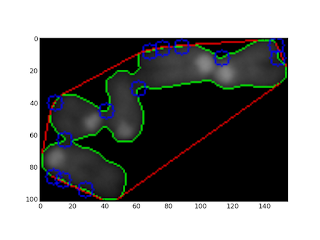## Friday, October 26, 2012

### Trying to separate touching mouse chromosomes

In the previous post, from a cluster of touching mouse chromosomes:

points belonging to negatively curved domains of a contour can be isolated :Yellow points belong to negatively curved domains of the contour.
To separate the chromosomes, it is tempting to cut between the closest pairs of points.

#### Let's make combination of pairs of points belonging to the contour:

Python provides itertools.combinations(); the closest pairs of points are in the set of the combinations of every pairs of points:

#### Path between two points belonging to the countour:

Each line shows the euclidian distance between two points, the four shortest lines correspond more or less to the contact domains between the chromosomes, cuting along them provides a way to separate the chromosomes.
It should be possible to do better by using a geodesic distance between the points, that is using a path inside the cluster of chromosomes. In the following example:

On the left, two paths (depending on the chosen pixel neighborhood) between the two blue points were overlaid on a binary image (background is set to 255 and the foreground to 0). The path were determined as follow using skimage 0.8dev:
graph.route_through_array(negbin ,p1,p2,fully_connected=False)
or
graph.route_through_array(negbin ,p1,p2,fully_connected=True)

• negbin: the negative binary image
• p1, p2: the two points figured in blue.

On the right the background (0) was set to 255 (white), the path (red) between the two points was determined by minimizing the "greyscale cost", that is through the valleys between the two points is the image is viewed as a greyscaled landscape.

All the paths can be determined (left), and then the paths can be sorted according to their cost (right). The four paths with the smallest cost are figured in red (left).

Download code.

or see bellow:

## Wednesday, October 24, 2012

### Contour : looking for negatively curved domains

A contour was previously explored with the convexity defects  algorithm implemented in opencv. Some highly curved domains  failed to be detected because of the contour shape.Curved domains missed by convexity defects detection

Using polygonal approximation imlemented in skimage 0.8 dev, positively curved domains as well as negatively curved domains on a contour can be sorted:Contour of a chromosomes cluster (blue), polygonal approximation (yellow). Red points: positive curvature. Yellow points: negative curvature.

From a segmented particle, a polygonal approximation of the contour is extracted.
Points of the polygon are used to compute an angle between two consecutive vectors (Here using the complex affix of vectors):

Positive angles correspond to convex domains and negative angles to concave domains.

The code has to be modified to filter angles according to a given range.

The code can be modified as follow to filter angles corresponding to curved domains:

• positive = table[(135>table[:,2]) & (table[:,2]>45)]
• negative = table[(-45>table[:,2]) & (table[:,2]>-135)]Curved domains on polygonal approximation of a chromosomes cluster contour.

Download code